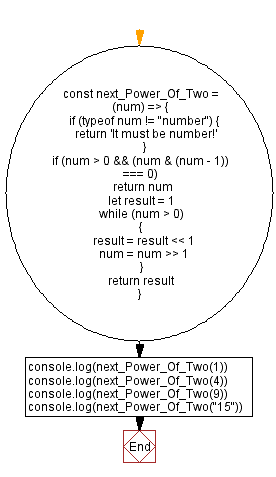# JavaScript - Next power of two of a given number

## JavaScript Bit Manipulation: Exercise-4 with Solution

Write a JavaScript program to find the next power of two of a given number.

A power of two is a number of the form 2n where n is an integer, that is, the result of exponentiation with number two as the base and integer n as the exponent.
The first ten powers of 2 for non-negative values of n are: 1, 2, 4, 8, 16, 32, 64, 128, 256, 512, ...

Test Data:
(1) -> 1
(4) -> 4
(9) -> 16
("15") -> "It must be number!"

Sample Solution:

JavaScript Code:

``````const next_Power_Of_Two = (num) => {
if (typeof num != "number") {
return 'It must be number!'
}

if (num > 0 && (num & (num - 1)) === 0)
return num
let result = 1

while (num > 0)
{
result = result << 1
num = num >> 1
}
return result
}
console.log(next_Power_Of_Two(1))
console.log(next_Power_Of_Two(4))
console.log(next_Power_Of_Two(9))
console.log(next_Power_Of_Two("15"))
```
```

Sample Output:

```1
4
16
It must be number!
```

Flowchart:Live Demo:

See the Pen javascript-bit-manipulation-exercise-4 by w3resource (@w3resource) on CodePen.

* To run the code mouse over on Result panel and click on 'RERUN' button.*

Improve this sample solution and post your code through Disqus

What is the difficulty level of this exercise?

Test your Programming skills with w3resource's quiz.

﻿